# British Money: Learning Pound and Pence Coins

British Money: Learning Pound and Pence Coins#### List of all British Pence Coins• Above are all of the coins used in the United Kingdom that have a value of less than one pound (100 pence).

#### List of the British Pound CoinsA one penny coin is the British coin of lowest value and is written as 1p for short.

A one pound coin is worth 100 pence and we write it with the symbol ‘£’.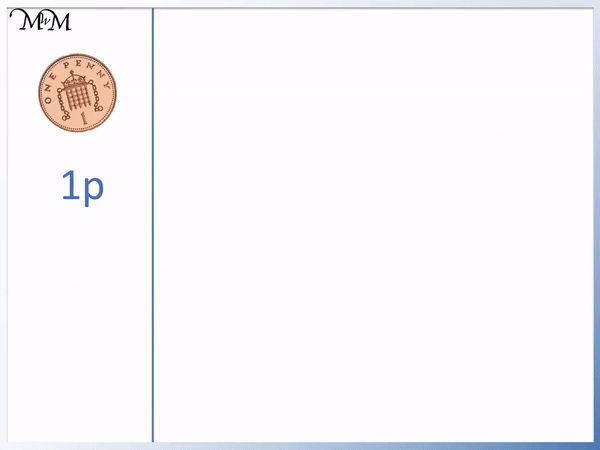• 1 ten Pence is worth the same as ten 1p coins.
• 10p is also worth the same as 5p + 5p.
• We could also make 10p from 5p + 2p + 2p + 1p.Supporting Lessons

#### Learning the British Pound Coins Video Lesson# Learning the British Coins

## Pictures of British Coins and their Values

In Britain, there are 6 different coins that are worth pennies and 2 different coins that are worth pounds.

Here is a list of all of the British Penny coins with their name and value shown below.In the UK, the coin with the lowest value is the 1 penny coin.

When we have more than 1 penny, we say that we have pence. So we have 2 pence, 5 pence, 10 pence, 20 pence and 50 pence coins.

The symbol for pence is ‘p’. This is because it is the first letter of the word pence.

We can write 1 penny as 1p, 2 pence as 2p, 5 pence as 5p, 10 pence as 10p, 20 pence as 20p and 50 pence as 50p.

In British money, the p is pronounced as ‘pee’ and is said after the number.

#### 1 Penny Coin

The penny coin is the lowest value British coin. The penny coin is also known as 1 pence and is written as 1p for short. This is pronounced as ‘1 pee’.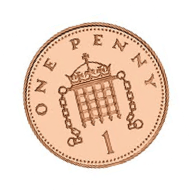#### 2 Pence Coin

Here is a 2 pence coin. We say pence now, rather than penny because 2 is more than 1. We only say penny for the 1 penny coin. Whenever we have a coin that is worth more than 1 penny, the word penny changed to pence.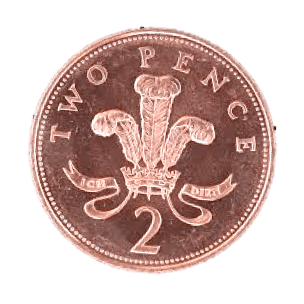We say that we have 2 pence, or 2p. This is pronounced as ‘2 pee’.2p is worth the same as two 1p coins.1 + 1 = 2 and so, 1p + 1p = 2p.

#### 5 Pence Coin

Here is a 5 pence coin.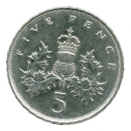5p is worth the same as five 1p coins.5 = 1 + 1 + 1 + 1 + 1 and so, 5p = 1p + 1p + 1p + 1p + 1p.

5p can be made from any combination of coins that add up to make 5p.

For example, 2 + 2 + 1 = 5 and so, 2p + 2p + 1p = 5p.Here we can see that 5p = 1p + 1p + 1p + 1p + 1p and for every two pennies we have, they can be replaced with a 2 pence coin.5p = 2p + 2p + 1p.

#### 10 Pence Coin

Here is a 10 pence coin, or 10p.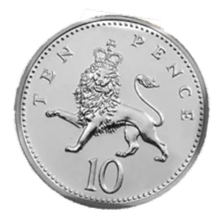10p is worth the same as 10 1p coins. It is much easier to carry 1 single 10p coin around instead of carrying ten pennies.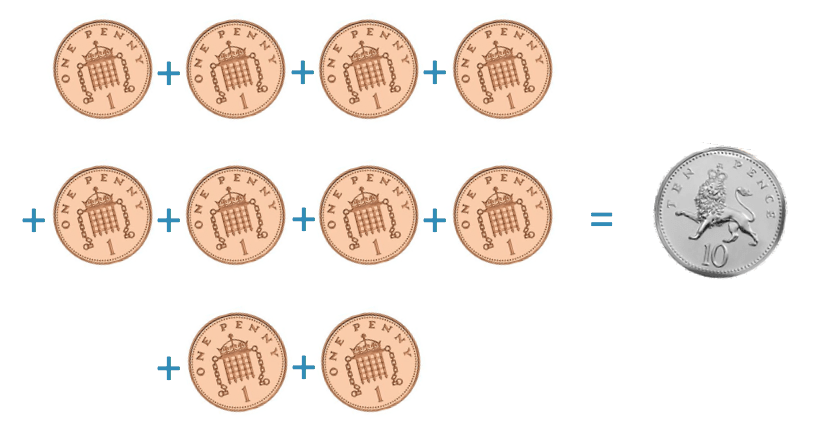5 + 5 = 10 and so, 5p + 5p = 10p.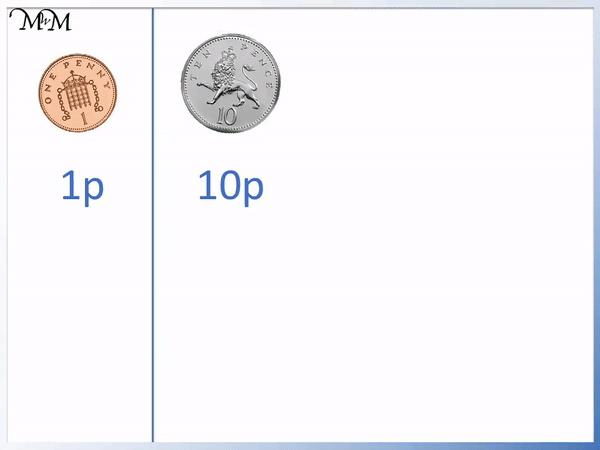Any combination of coins that add to make ten pence can be replaced with a ten pence coin.

For example, 5 + 2 + 2 + 1 = 10 and so ten pence can be made from a 5p, 2p, 2p and a 1p coin.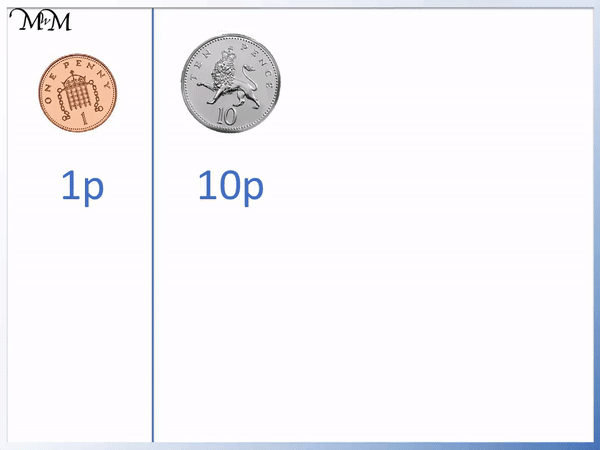#### 20 Pence Coin

Here is the 20 pence coin.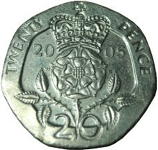20p is worth the same as 10p + 10p.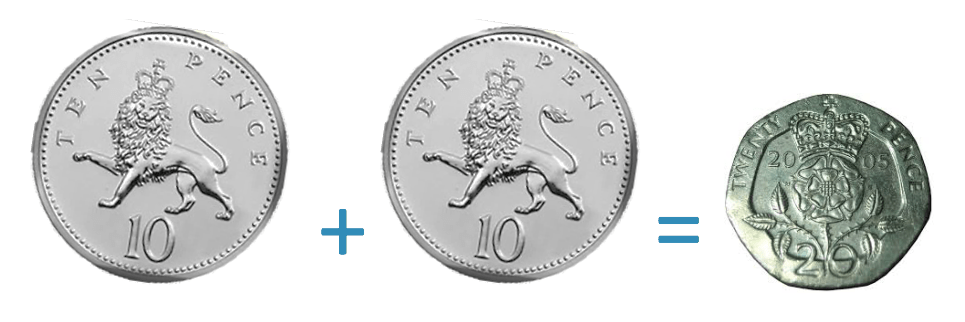#### 50 Pence Coin

Here is a 50 pence coin. The 50 pence coin is the largest coin in terms of size. The 50 pence coin is the pence coin with the largest value. There is no other coin that is worth more than a 50p apart from the pound coins.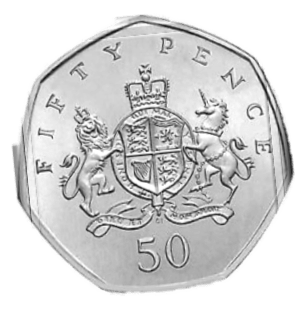Any combination of coins that add to make 50 are worth the same as a 50 pence piece.

For example, 20 + 20 + 10 = 50 and so a 50 pence coin is worth the same as 20p + 20p + 10p.## British Pound Coins

Here are the 2 different coins that are worth £1 and £2.1 pound is worth 100 pennies. Instead of 100 1p coins, a £1 coin can be used. Any combination of coins that add up to 100 pence can be replaced with a pound coin.

£1 = 100p

There are many different ways that we can make £1. We can make a pound by using any combination of coins that add up to 100p.

The £ symbol is the pound symbol. £1 is actually said with the number first and then the word pound. So we say £1 as ‘one pound’. We say £2 as ‘two pounds’.

For example, we can use two 50p coins to make a pound.This is because 50p + 50p = 100p.100p = £1

Another way to make £1 is to use a 50p coin, two 20p coins and a 10p coin.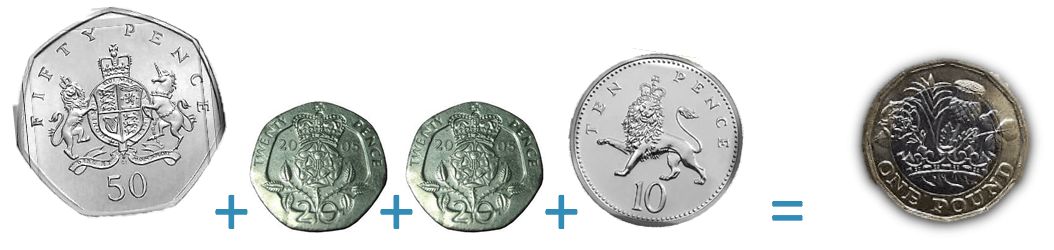50p + 20p + 20p + 10p = 100p

100p = £1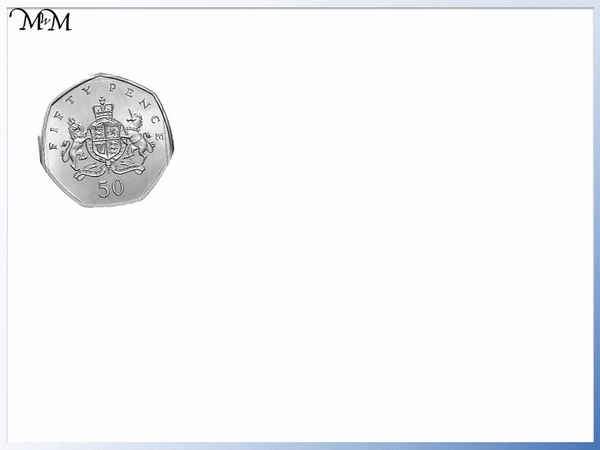Here we have a combination of coins that add to make £1. We can keep adding the total together until we have 100 pence.

Here is a £2 coin.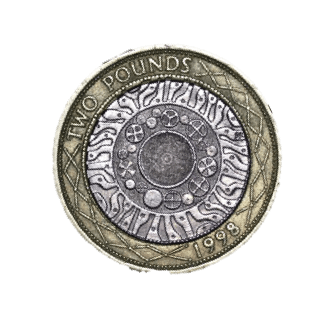The 2 pound coin is worth the same as 2 pound coins combined. A 2 pound coin is worth 200 pence.Each pound is worth 100p and so 100p + 100p = 200p.£2 = 200p and so any combination of coins that adds to 200 pence can be replaced with a £2 coin.

For example we have £1 + 50p + 50p.The £1 is worth 100p. 100p + 50p + 50p = 200p, which is the same value as £2.It is helpful to group combinations of coins that make £1. Counting pounds can be easier than counting pennies with larger amounts of money.

In this example, the two 50p coins can be combined to make £1.

£1 + £2 + £1 = £4.Now try our lesson Counting British Coins: Adding Pounds and Pence where we learn how to add different groups of British coins.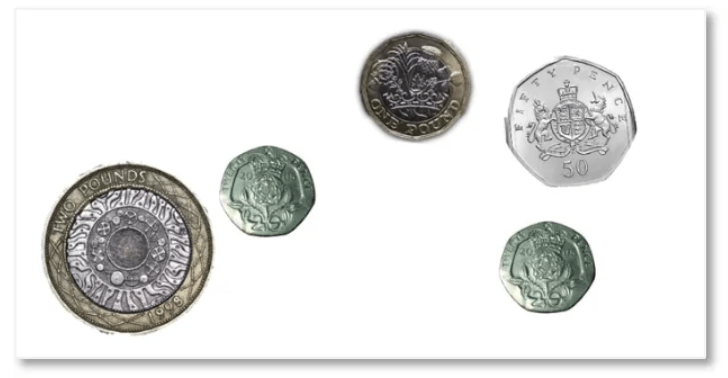error: Content is protected !!